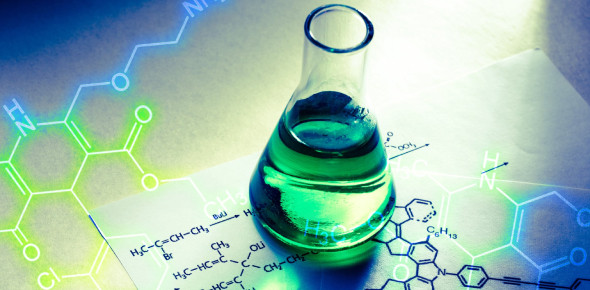# Basic Chemistry Test: MCQ Trivia Quiz!

9 Questions | Attempts: 10324
ShareSettingsWould you like to take this basic chemistry test? This quiz might be the one for you. Basic chemistry is the science and examination of matter, including its elements, composition, and reactivity. Chemistry pertains to everything that can be taken, from the little variables to complex structures. The atom and molecules are the basic units or components of chemistry. This basic chemistry quiz has the right formula to help you understand and even appreciate this subject.

• 1.
Like the one below, chemical equations must be balanced. This shows that
• A.

The atoms on the reactant side are changed into different atoms on the products side.

• B.

The chemical reaction is not reversible.

• C.

The chemical reaction is reversible.

• D.

The number of atoms in the reactants is equal to the number of atoms in the product.

• 2.
In the chemical reaction between hydrochloric acid () and calcium carbonate (), which of the following elements cannot be part of the products?
• A.

Carbon (C)

• B.

Nitrogen (N)

• C.

Chlorine (Cl)

• D.

Hydrogen (H)

• 3.
Which of the following is an example of a chemical reaction?
• A.

Tearing paper

• B.

Evaporating water

• C.

Baking a cake

• D.

Coloring a picture

• 4.
Lithium, a highly reactive metal, is a member of the alkali metals. Use the periodic table to determine which element would have chemical properties that are similar to lithium.
• A.

Sodium

• B.

Carbon

• C.

Oxygen

• D.

Mercury

• 5.
Which element on the periodic table has physical properties MOST similar to the physical properties of chlorine?
• A.

Sodium

• B.

Oxygen

• C.

Carbon

• D.

Florine

• 6.
Which BEST describes the form an element in Group 18 on the periodic table takes at room temperature?
• A.

A shiny solid

• B.

A dark liquid

• C.

A gas

• D.

A clear mix of liquid and solid

• 7.
Xenon is a gas at room temperature. Because of the arrangement of the periodic table, which other element can BEST be predicted to share this physical property?
• A.

Potassium

• B.

Rubidium

• C.

Iodine

• D.

Krypton

• 8.
Which of the following determines the identity of an element?
• A.

Atomic number

• B.

Mass number

• C.

Atomic mass

• D.

Overall change

• 9.
Which element has a valence electron configuration most similar to that of bromine (Br)?
• A.

Zinc (Zn)

• B.

Sulfur (S)

• C.

Fluorine (F)

• D.

Krypton (Kr)

## Related TopicsBack to top
×

Wait!
Here's an interesting quiz for you.